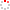NSSC OpenIR
 Superconvergence analysis of two-grid FEM for Maxwell's equations with a thermal effect Alternative Title WOS:000531076600009;20201008258637 Yao, C. H.; Li, F. R.; Zhao, Y. M. Source Publication COMPUTERS & MATHEMATICS WITH APPLICATIONS2020 Volume 79Issue:12Pages:3378-3393 DOI 10.1016/j.camwa.2020.02.001 ISSN 0898-1221 Language 英语 Keyword Maxwell's equation Thermal effect Nedelec element Two-grid algorithm Superconvergence FINITE-ELEMENT-METHOD H-PSI FORMULATION WAVE-PROPAGATION HOMOGENIZATION MODEL Abstract Based on two-grid algorithm, we develop the superconvergence analysis of fully-discrete scheme with the lowest-order Nedelec element and Crank-Nicolson scheme for the magneto-heat coupling model, which is also considered as Maxwell's equations with a thermal effect. Then, main process of numerical analysis has two parts: On one hand, we utilize Newton-type Taylor expansion on superconvergent solutions for those nonlinear terms on coarse mesh, differing from the numerical solution, which makes our given two-grid method successful. On the other hand, we prove the solutions on the fine mesh to be higher accuracy by the postprocessing interpolation technique. Such a design is conducive to improving the computational accuracy in space and decreasing time consumption simultaneously. By employing the skill, we can obtain the convergent rate of O(Delta t(2) + h(2 )+ H-3 ), which means that the space mesh size should satisfy h = O(H-3/2). Finally, the numerical example is presented to verify the theoretical results. (C) 2020 Elsevier Ltd. All rights reserved. Indexed By SCI ; EI Citation statistics Document Type 期刊论文 Identifier http://ir.nssc.ac.cn/handle/122/7722 Collection 中国科学院国家空间科学中心 Affiliation 1.[Yao, C. H.2.Li, F. R.] Zhengzhou Univ, Sch Math & Stat, Zhengzhou 450001, Peoples R China3.[Yao, C. H.] Chinese Acad Sci, State Key Lab Space Weather, Beijing 100190, Peoples R China4.[Zhao, Y. M.] Xuchang Univ, Sch Math & Stat, Xuchang 461000, Peoples R China Recommended CitationGB/T 7714 Yao, C. H.,Li, F. R.,Zhao, Y. M.. Superconvergence analysis of two-grid FEM for Maxwell's equations with a thermal effect[J]. COMPUTERS & MATHEMATICS WITH APPLICATIONS,2020,79(12):3378-3393. APA Yao, C. H.,Li, F. R.,&Zhao, Y. M..(2020).Superconvergence analysis of two-grid FEM for Maxwell's equations with a thermal effect.COMPUTERS & MATHEMATICS WITH APPLICATIONS,79(12),3378-3393. MLA Yao, C. H.,et al."Superconvergence analysis of two-grid FEM for Maxwell's equations with a thermal effect".COMPUTERS & MATHEMATICS WITH APPLICATIONS 79.12(2020):3378-3393.
 Files in This Item: There are no files associated with this item.
 Related Services Recommend this item Bookmark Usage statistics Export to Endnote Google Scholar Similar articles in Google Scholar [Yao, C. H.]'s Articles [Li, F. R.]'s Articles [Zhao, Y. M.]'s Articles Baidu academic Similar articles in Baidu academic [Yao, C. H.]'s Articles [Li, F. R.]'s Articles [Zhao, Y. M.]'s Articles Bing Scholar Similar articles in Bing Scholar [Yao, C. H.]'s Articles [Li, F. R.]'s Articles [Zhao, Y. M.]'s Articles Terms of Use No data! Social Bookmark/Share